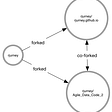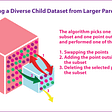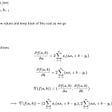# y = e^(b0 + b1*x) / (1 + e^(b0 + b1*x))

• Logit = ln(P(X)/(1-P(X)))
• ODDS = P(X)/(1-P(X)
• The output variables must always be binary because Logistic Regression assumes output as Binary.
• Logistic Regression assumes there exist no noise ,so remove all the noise from data.
• Logistic Regression assumes Gaussian Distribution and there exist a linear relationship between our input and output variable.
• Remove all the correlated input variables. If correlated input variables occur model can highly overfit.

--

--

--

## More from Akshar Rastogi

Love podcasts or audiobooks? Learn on the go with our new app.

## Introduction to Movie Recommendation System for Beginners## Part 8: Review of Logistic Regression and its Implementation in Tensorflow / Keras## Smart rankings at Farfetch: 101## How diversity could solve for algorithmic bias## How to Build a Smart Vision-Based Sudoku Solver## Creating new “art” works by integrating Creative Adversarial Network (CAN) and Neural Style…## NLP Pipeline Step-by-Step## Understanding Decision Trees ID3 Algorithm |Machine Learning## A take on implementing Linear Regression from scratch — Part 1